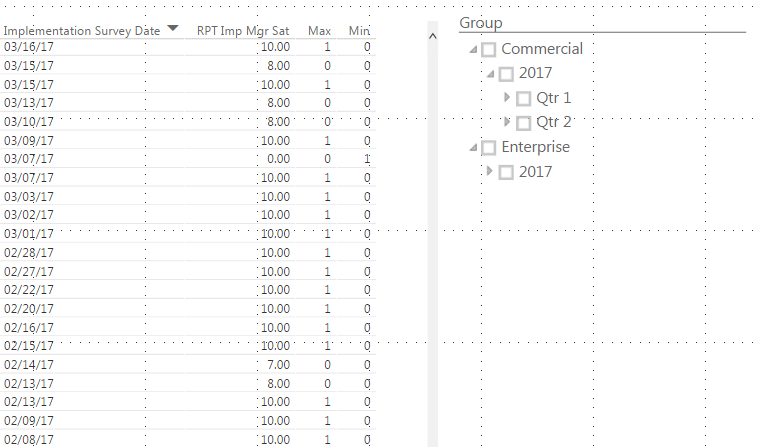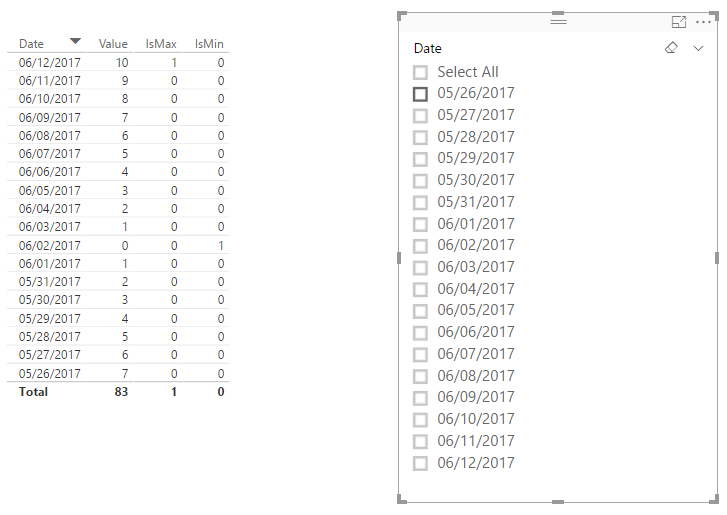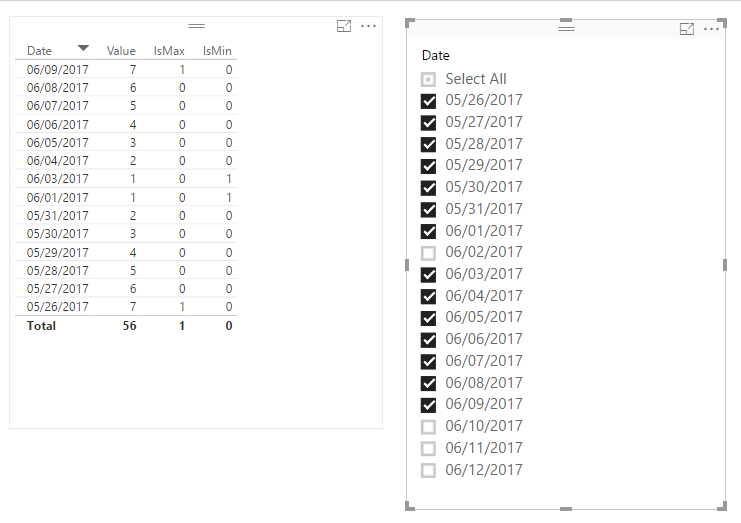cancel
Showing results for
Did you mean:Helper I

## getting the MIN or MAX value after filtering in visualization

I have a table that shows values from 1 to 10

and I have a date column as well and group column

I want to show the values excluding the dynamic maximum value and excluding the dynamic minimum value.

I created an identifier column that identifies the max value as 1, if it's not, its a 0.

I also have created an identifier column that identifies the min value as 1, if it's not, its a 0.

the problem I am having is when I use filters.

for example, I want to see only the values from the 1st quarter only of one group.

the result is not showing the minimum since it thinks that it is not the minimum, it still sees the true minimum without the filters.

without filter, it is showing the minwith filter, it's not showing the min based on the filter1 ACCEPTED SOLUTIONMicrosoft

Not like measures, calculate columns/tables are computed during database processing(e.g. data refresh) and then stored in the model, they do not response to user selections on the report.

So it is not possible to create a calculate column/table can change dynamically with user selections on the report.

Normally, we can create measures instead. The formulas below are for your reference.```IsMax =
VAR maxValue =
CALCULATE ( MAX ( Table1[Value] ), ALLSELECTED ( Table1 ) )
VAR currentValue =
MAX ( Table1[Value] )
RETURN
IF ( currentValue = maxValue, 1, 0 )
```
```IsMin =
VAR minValue =
CALCULATE ( MIN ( Table1[Value] ), ALLSELECTED ( Table1 ) )
VAR currentValue =
MAX ( Table1[Value] )
RETURN
IF ( currentValue = minValue, 1, 0 )```

1. Result without filter.2, Result with filter.Regards

3 REPLIES 3Microsoft

Not like measures, calculate columns/tables are computed during database processing(e.g. data refresh) and then stored in the model, they do not response to user selections on the report.

So it is not possible to create a calculate column/table can change dynamically with user selections on the report.

Normally, we can create measures instead. The formulas below are for your reference.```IsMax =
VAR maxValue =
CALCULATE ( MAX ( Table1[Value] ), ALLSELECTED ( Table1 ) )
VAR currentValue =
MAX ( Table1[Value] )
RETURN
IF ( currentValue = maxValue, 1, 0 )
```
```IsMin =
VAR minValue =
CALCULATE ( MIN ( Table1[Value] ), ALLSELECTED ( Table1 ) )
VAR currentValue =
MAX ( Table1[Value] )
RETURN
IF ( currentValue = minValue, 1, 0 )```

1. Result without filter.2, Result with filter.RegardsHelper I

Hi v-ljerr-msft -

I really appreciate it.

I am just having problems incorporating your DAX formula.

I have updated Table1[value] with what I have on file as well.

But, I am seeing the currentValue as "grey" when I put the formula in.

compared to maxValue which is green.

I am also seeing the error message, the syntax for 'VAR' is incorrectMicrosoft

Could you post the DAX formula you're using which is not working? So that I can help further investigate on the issue. It's better to share a sample pbix file which can reproduce the issue. You can upload it to OneDrive or Dropbox and post the link here. Do mask sensitive data before uploading.Regards